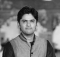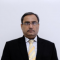## Python Pandas - Extract the Number of seconds for each element from TimeDeltaIndexPublished on 19-Oct-2021 10:54:01
To extract the Number of seconds for each element from TimeDeltaIndex object, use the TimedeltaIndex.seconds propertyAt first, import the required libraries −import pandas as pdCreate a TimeDeltaIndex object. We have set the timedelta-like data using the 'data' parameter as well −tdIndex = pd.TimedeltaIndex(data =['10 day 5h 2 min 35s 3us ... Read More

## Program to find out the cost to merge an array of integers into a single value in C++Published on 19-Oct-2021 10:53:33
Suppose we are given an array arr that contains n positive integer numbers. We are also given an integer number j. The task we have to perform is to merge j numbers into a single number by adding them. The cost of merging is equal to the addition of the ... Read More

## Program to find length of longest sublist with value range condition in PythonPublished on 19-Oct-2021 10:49:30
Suppose we have a list of numbers called nums, we have to find the length of the longest sublist where 2 * (minimum of sublist) > (maximum of sublist).So, if the input is like nums = [10, 2, 6, 6, 4, 4], then the output will be 4, because the ... Read More

## Program to find length of longest repeating substring in a string in PythonPublished on 19-Oct-2021 10:46:07
Suppose we have a lowercase string s, we have to find the length of the longest substring that occurs at least twice in s. If we cannot find such string, return 0.So, if the input is like s = "abdgoalputabdtypeabd", then the output will be 3, because the longest substring ... Read More

## Program to find length longest prefix sequence of a word array in PythonPublished on 19-Oct-2021 10:43:05
Suppose we have a list of words called w, with lowercase strings. We have to find the length of the longest sequence of w where each previous word is the prefix of the next word and the next word has just one new character appended.So, if the input is like ... Read More

## Program to find length of longest matrix path length in PythonPublished on 19-Oct-2021 10:40:38
Suppose we have a binary matrix, where 0 indicates empty cell and 1 indicates wall. We can start at any empty cell on first row and want to end up on any empty cell on the last row. We can move left, right, or down, we have to find the ... Read More

## Program to find length of longest contiguously strictly increasing sublist after removal in PythonPublished on 19-Oct-2021 10:37:04
Suppose we have a list of numbers called nums, we have to find the maximum length of a contiguous strictly increasing sublist. We are allowed to remove at most single element from the list.So, if the input is like nums = [35, 5, 6, 7, 8, 9, 12, 11, 26], ... Read More

## Program to find length of longest consecutively increasing substring in PythonPublished on 19-Oct-2021 10:34:27
Suppose we have a lowercase string s. This contains English letters as well as "?" Symbol. For each "?" we must either remove it or replace it with any lowercase letter. We have to find the length of the longest consecutively increasing substring that starts with letter "a".So, if the ... Read More

## Program to find length of longest consecutive sublist with unique elements in PythonPublished on 19-Oct-2021 10:32:05
Suppose we have a list of numbers called nums, where all elements are unique. We have to find the length of the longest sublist that contains consecutive elements.So, if the input is like nums = [3, 6, 7, 5, 4, 9], then the output will be 5, because the sublist ... Read More

## Program to find longest consecutive run of 1s in binary form of n in PythonPublished on 19-Oct-2021 10:28:26
Suppose we have a non-negative value n, we have to find the length of the longest consecutive run of 1s in its binary representation.So, if the input is like n = 1469, then the output will be 4, because binary representation of 156 is "10110111101", so there are four consecutive ... Read More